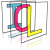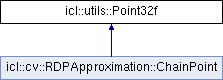Image Component Library (ICL)
icl::utils::Point32f Class Reference

Single precission 3D Vectors Point class of the ICL. More...

`#include <Point32f.h>`

Inheritance diagram for icl::utils::Point32f:## Public Member Functions

Point32f ()
default constructor More...

Point32f (const Point32f &p)
deep copy of a Point More...

Point32f (float x, float y)
create a special point More...

Point32f (const Point &p)
craete a point by a given interger point More...

bool isNull () const
checks wether the object instance is null, i.e. all elements are zero More...

bool operator== (const Point32f &s) const
checks if two points are equal More...

bool operator!= (const Point32f &s) const
checks if two points are not equal More...

Point32f operator+ (const Point32f &s) const
adds two Points as vectors More...

Point32f operator- (const Point32f &s) const
substracts two Point32fs as vectors More...

Point32f operator * (double d) const
scales a Point32fs variables with a scalar value More...

Point32foperator+= (const Point32f &s)

Point32foperator-= (const Point32f &s)
Substacts another Point32f inplace. More...

Point32foperator *= (double d)
scales the Point32f inplace with a scalar More...

Point32f transform (double xfac, double yfac) const
transforms the point by element-wise scaling More...

float distanceTo (const Point32f &p) const
returns the euclidian distance to another point More...

bool inTriangle (const utils::Point32f &v1, const utils::Point32f &v2, const utils::Point32f &v3) const
Checks whether the given point p lies within the triangle defined by v1,v2 and v3. More...

float norm (float p=2) const
returns the p-norm of the 2D Vector More...

Point32fnormalize ()
normalized this 2D vector to length=1; More...

Point32f normalized () const
returns a normalized version of this Point More...

float & operator[] (int i)
index based interface (returns i?y:x) More...

const float & operator[] (int i) const
index based interface, const (returns i?y:x) More...

## Public Attributes

float x
x position of this point More...

float y
y position of this point More...

## Static Public Attributes

static const Point32f null
null Point is x=0, y=0 More...

## Detailed Description

Single precission 3D Vectors Point class of the ICL.

## ◆ Point32f() [1/4]

 icl::utils::Point32f::Point32f ( )
inline

default constructor

## ◆ Point32f() [2/4]

 icl::utils::Point32f::Point32f ( const Point32f & p )
inline

deep copy of a Point

## ◆ Point32f() [3/4]

 icl::utils::Point32f::Point32f ( float x, float y )
inline

create a special point

## ◆ Point32f() [4/4]

 icl::utils::Point32f::Point32f ( const Point & p )
inline

craete a point by a given interger point

## ◆ distanceTo()

 float icl::utils::Point32f::distanceTo ( const Point32f & p ) const

returns the euclidian distance to another point

## ◆ inTriangle()

 bool icl::utils::Point32f::inTriangle ( const utils::Point32f & v1, const utils::Point32f & v2, const utils::Point32f & v3 ) const

Checks whether the given point p lies within the triangle defined by v1,v2 and v3.

## ◆ isNull()

 bool icl::utils::Point32f::isNull ( ) const
inline

checks wether the object instance is null, i.e. all elements are zero

## ◆ norm()

 float icl::utils::Point32f::norm ( float p = `2` ) const

returns the p-norm of the 2D Vector

• p = 0 -> 2
• p = 1 -> city block norm x+y
• p = 2 -> euclidian norm
• p->inf -> infinity norm
Parameters
 p chooses the norm
Returns
norm value

## ◆ normalize()

 Point32f& icl::utils::Point32f::normalize ( )
inline

normalized this 2D vector to length=1;

uses the euclidian norm! @ return a reference to (this) normalized

## ◆ normalized()

 Point32f icl::utils::Point32f::normalized ( ) const
inline

returns a normalized version of this Point

Returns
normalized vec

## ◆ operator *()

 Point32f icl::utils::Point32f::operator * ( double d ) const
inline

scales a Point32fs variables with a scalar value

## ◆ operator *=()

 Point32f& icl::utils::Point32f::operator *= ( double d )
inline

scales the Point32f inplace with a scalar

## ◆ operator!=()

 bool icl::utils::Point32f::operator!= ( const Point32f & s ) const
inline

checks if two points are not equal

## ◆ operator+()

 Point32f icl::utils::Point32f::operator+ ( const Point32f & s ) const
inline

## ◆ operator+=()

 Point32f& icl::utils::Point32f::operator+= ( const Point32f & s )
inline

## ◆ operator-()

 Point32f icl::utils::Point32f::operator- ( const Point32f & s ) const
inline

substracts two Point32fs as vectors

## ◆ operator-=()

 Point32f& icl::utils::Point32f::operator-= ( const Point32f & s )
inline

Substacts another Point32f inplace.

## ◆ operator==()

 bool icl::utils::Point32f::operator== ( const Point32f & s ) const
inline

checks if two points are equal

## ◆ operator[]() [1/2]

 float& icl::utils::Point32f::operator[] ( int i )
inline

index based interface (returns i?y:x)

## ◆ operator[]() [2/2]

 const float& icl::utils::Point32f::operator[] ( int i ) const
inline

index based interface, const (returns i?y:x)

## ◆ transform()

 Point32f icl::utils::Point32f::transform ( double xfac, double yfac ) const
inline

transforms the point by element-wise scaling

## ◆ null

 const Point32f icl::utils::Point32f::null
static

null Point is x=0, y=0

## ◆ x

 float icl::utils::Point32f::x

x position of this point

## ◆ y

 float icl::utils::Point32f::y

y position of this point

The documentation for this class was generated from the following file:
• /Users/alneuman/vm/icl/ICLUtils/src/ICLUtils/Point32f.h